• 单变量线性回归 (Linear regression with one variable)：只有一个自变量；
• 多变量线性回归 (Linear regression with multiple variable)：有多个自变量。

## ✎模型表达 (Model representation)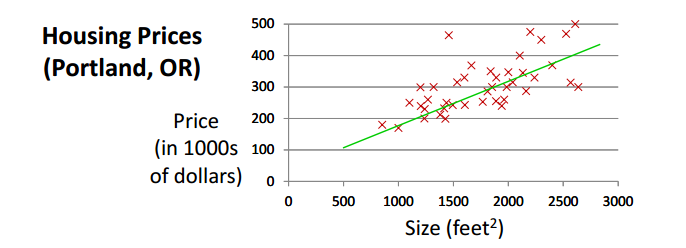• m = Number of training examples （训练样例的数量）
• x’s = “input” variable / features （输入变量 / 特征）
• y’s = “output” variable / “target” variable （输出变量 / 目标变量）
• (x,y) - one training example （一个训练样例）
• (x(i),y(i)) - ith training example （第 i 个训练样例）
• h (hypothesis) - hθ(x) = θ01x （学习算法的解决方案或函数，将 x’s 映射到 y’s）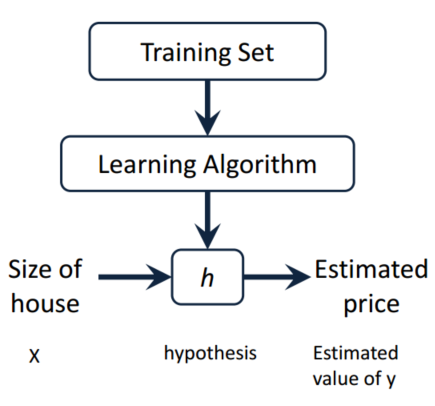## ✎损失函数 (Cost function)

Hypothesis: hθ(x) = θ01x , 参数 θi’s 如何选择？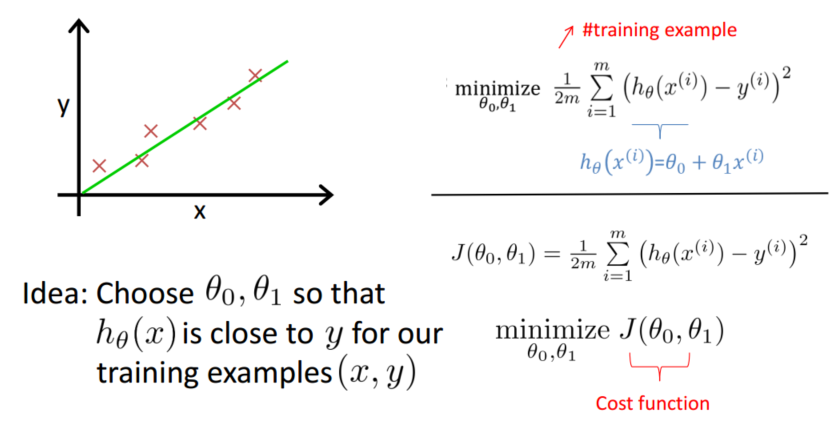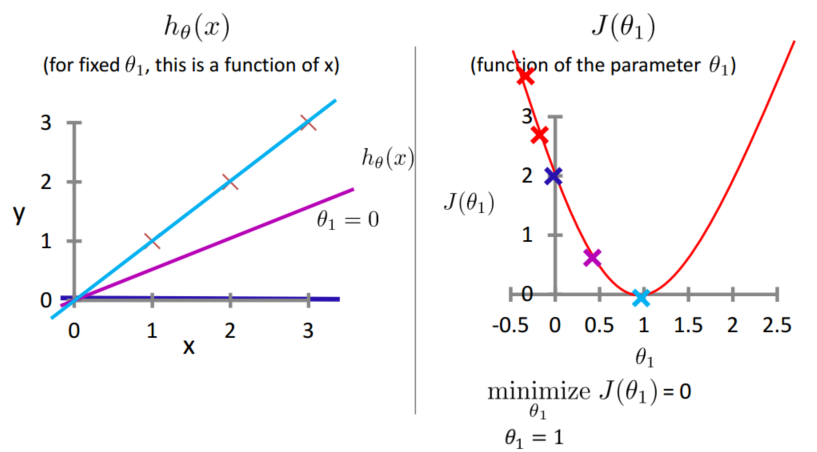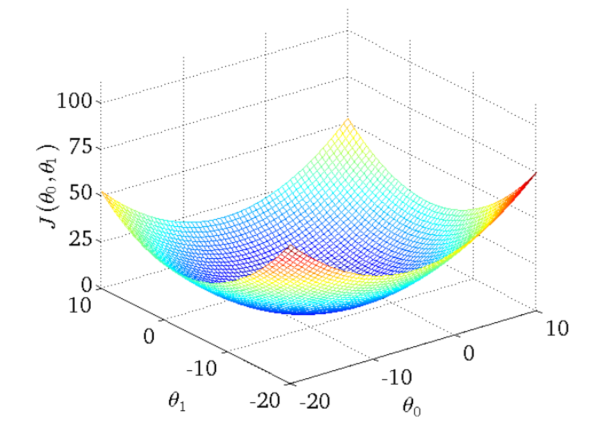0%